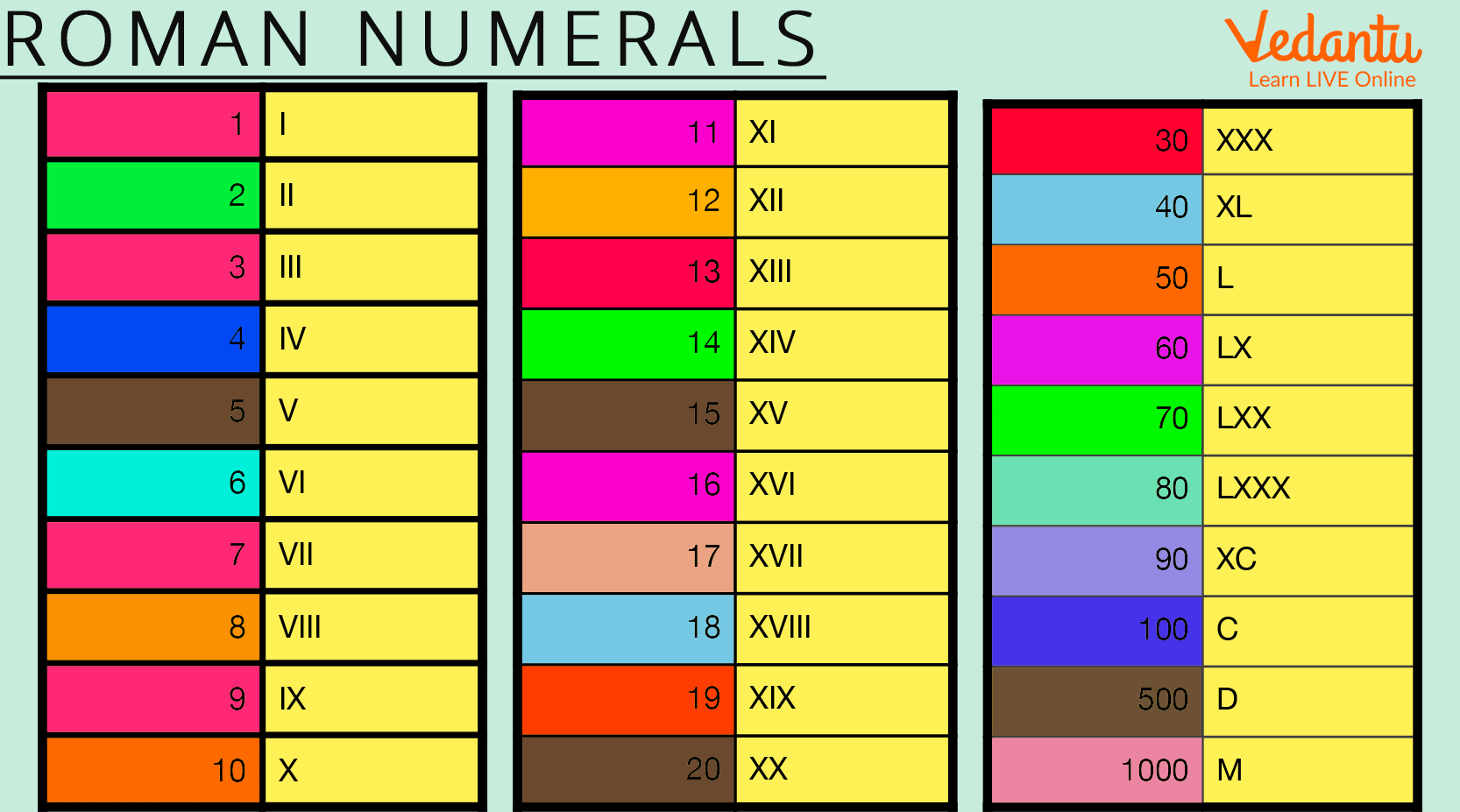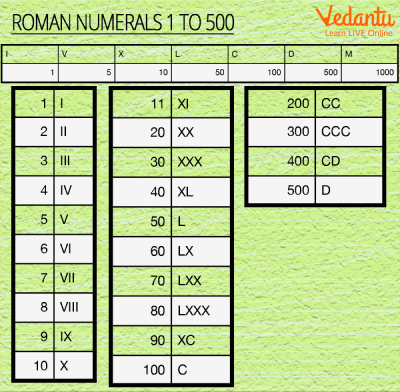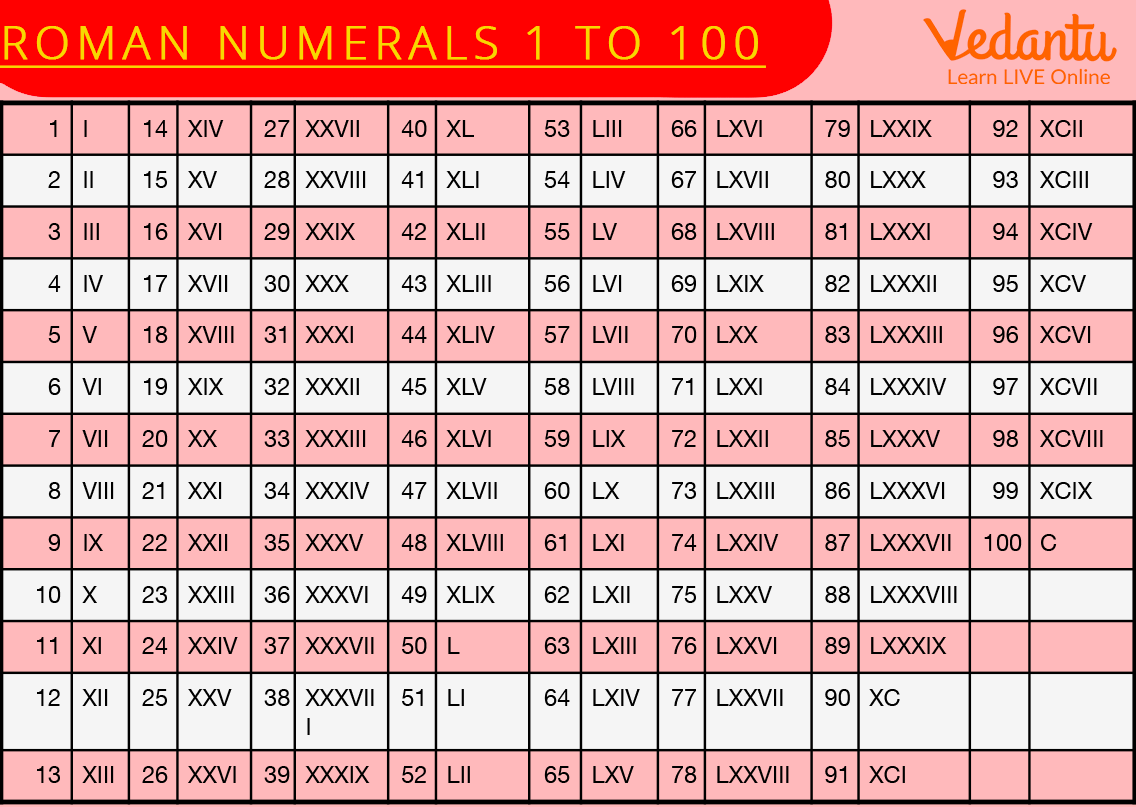Courses
Courses for Kids
Free study material
Free LIVE classes
More

# Roman Numbers From 1 to 500## Introduction to Roman Numerals

Roman numbers are defined as a way of representing numbers in the Roman numerical system: I = 1, V=5, L=50, and so on. It is a numerical representation of digits as in the ancient Roman system.

We can also define Roman numbers as a number system that originated in ancient Rome. There are no zeros in this system. This system is still used in many places. In Roman numerals, alphabets are used to represent the fixed positive numbers, these are written as follows:Roman Numbers

In this system, a letter placed after another of greater value adds whereas a letter placed before another of greater value subtracts.

We all have come across different types of fonts and different ways in which digits are written. Some addresses are written including numbers X and VII, and even sections or classes in a school are written in such patterns or ways. After 10, the Roman numerals are followed by XI for 11, XII for 12….till XX for 20. The most common Roman numbers are given in the table below:Roman Numerals

In this article, Roman numerals have been discussed in detail. A chart containing Roman numbers from 1 to 500 is also shown in the article below.

## Roman Numerals (1 to 500)

Below is the chart for Roman numerals that shows the Roman numerals from 1 to 500. Using this chart, we can easily write numbers and can understand the concept of Roman numbers.Roman Numerals

## Rules to Write Roman Numerals

There are certain rules to be followed while presenting a number in Roman numerals which are as follows:

• The value of the symbol is added to itself as many times as it is repeated. For example II = 2, XX = 20, XXX = 30.

• Symbols such as V, L, and D are never repeated and are never subtracted.

• When a symbol of a smaller value appears after a symbol of greater value, its value will be added for example-  VI = V + I =5 + 1 = 6.

• When a symbol of smaller value appears before a symbol of greater value symbol, its value will be subtracted for example IX = X - I = 10 -1 = 9

• The symbol I can be subtracted from V and X only and symbol X can be subtracted from symbols L, M, and C only.

Also, we can clearly understand that there is no requirement of writing zeros to represent 50 or 100; we can just write L and C. Thus, these letters are considered to be very easy and indicate the entire number system.Content of Book in RomanOne to Hundred Roman Number

## Interesting Facts About Roman Numerals

Roman numerals are used in many places, and things such as on the faces of watches and clocks, in calendars some dates are written in Roman numerals, and roll numbers are assigned to students in Roman numerals in schools. Also in books, the numbering of chapters follows the Roman numerals. They are also used in astronomy to designate the moon's position.The Face of a Wall Clock in  Roman Numbers

• Roman numerals are also used in fractions, which are uncia.

• Kings and Queens are named after Roman numerals such as Henry VII.

• The Romans would sometimes use Roman numerals as the days of the week.

• Around the 8th to 9th century B.C.E, Roman numerals were discovered.

• Roman numerals are also used in the National Football League in the numbering system of the Super Bowl.

## Solved Examples

Q 1. Write 1774 in Roman Numerals.

Ans: So let us understand how we can write this:

First of all, we need to break 1774 into 1000, 700, 70, and 4 and then do each conversion

As we know 1000 = M, 700 = DCC,70 = LXX,4 = IV

1000 + 700 + 70 + 4 = 1774,

Hence, 1774 = MDCCLXXVI

Q 2. Write 800 in Roman numerals.

Ans: First we need to break 800

800 = 500 + 100 + 100 + 100

As we know that 500 = D and 100 = C

Therefore, 800 = DCCC

## Practise Questions

Q 1. Write the following in roman numbers.

• 450

• 600

• 500

• 920

Ans: 450 = CDL , 600 = DC, 500 = D, 920 = CMXX

## Summary

Historians believed that the Roman numerals played a critical part during the creation of architecture. Also, their importance cannot be underestimated as it enabled Romans to put a price on their goods and services. Also, there are countless theories based on Roman numerals.

Last updated date: 21st Sep 2023
Total views: 121.5k
Views today: 2.21k

## FAQs on Roman Numbers From 1 to 500

1. Write 69 in Roman numerals.

69 in Roman numerals can be written as follows:  69= 60+9

=  (50+10) + (10-1)

=  LX + IX

69=  LXIX

To write a number in Roman numerals, we need to follow certain steps. Some fixed rules and regulations have been formulated to write down digits in the correct way. One needs to follow the symbolic representation of digits, and these are fixed in nature. As in the above case, we have written 50 as L, whereas 10 is written as X and 10-1 =9 which is written as LX. By joining the numeric representation, we can conclude to write 69 as LXIX. Thus, Roman numerals can be written if one knows or has knowledge about the rules.

2. What are Roman numerals?

Roman numerals are letters that the Romans used to count. Roman numerals are a numeral system that originated in ancient Rome. Numbers in this system are represented by combinations of letters and alphabets; also they represent a fixed integer value. Roman numbers are usually written in order from largest to smallest and from left to right and are still an important part of the modern system. Roman numbers also comprise Latin alphabets. So, we can say that Roman numerals have made Mathematics more interesting.

3. What are some uses of the Roman numerals?

Roman numerals are quite helpful. There are lots of things, and places, where Roman numerals are still used, such as Roman numerals, are used to count, they are used to name kings and queens, they are used to write the dates of things, they are used on the faces of wall clocks and wrist watches. While in books, the content is written using Roman numerals. Moreover, in schools, the classes are represented in Roman numerals. Many generations have a family name that has been passed down and a Roman numeral to signify the family member.